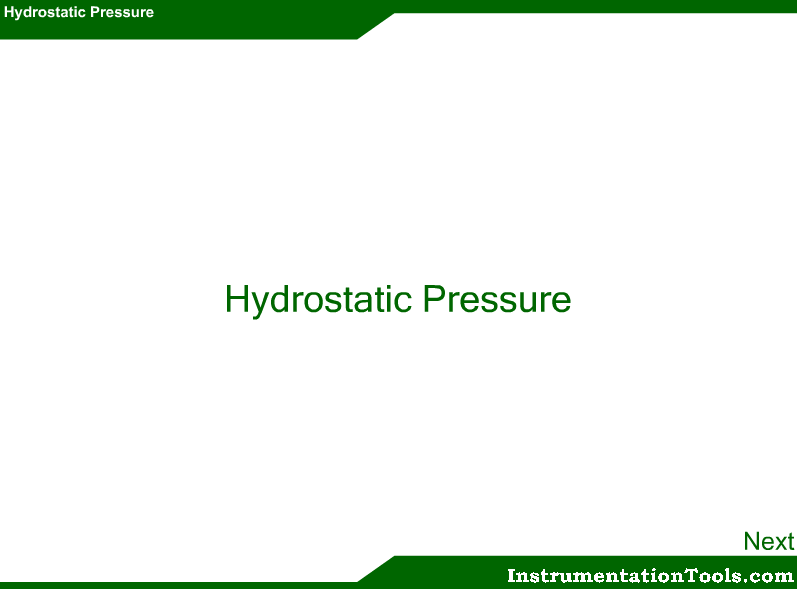# Hydrostatic Level Measurement

Hydrostatic Level Measurement

This method rely on the pressure of the measured liquid head to provide level indication. The pressure exerted by a column of liquid is equal to the height of the column, h, times the specific gravity of the liquid, sg. (P=h⋅(sg), where sg=ρ⋅g). Thus, from this we can calculate the level of the liquid in a vessel or tank, (i.e. the h), if we know the pressure at the bottom of the tank and the specific gravity of the liquid, sg.By referring to the figure above, the weight of one cubic foot container of water is seen to be 62.4 pounds (from its weight), and this force is exerted over the surface of the bottom of the container.

Area, A = 12 in × 12 in = 144 in²     (Since 1 ft = 12 in)

Pressure exerted on the area, P = F/A = 62.4 lbs/144 in² = 0.433 lbs/in²

We calculate equivalent level value based on the measured pressure and pressure directly proportional to the level. if pressure increases that means level in the column/tank increase and vice-versa.

Hydrostatic Level Measurement Animation1.